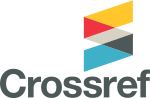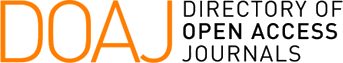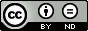### A Comparison of two Parallel Iterative Algorithms for Solving Path Problems

Robert Manger

#### Abstract

Path problems are a family of optimization and enumeration problems posed on a directed graph. General algorithms for solving path problems can be designed as counterparts of the traditional iterative methods for solving linear systems. In this paper two parallel iterative Gauss-Seidel-like algorithms for solving path problems arc compared. Theoretical results are listed, which estimate the computational complexity of both algorithms. Experiments are presented, where the algorithms have been tested on randomly generated graphs and with different numbers of available processors. Some situations are identified, where one of the algorithms becomes superior to the other.

#### Keywords

directed graphs, path problems, parallel algorithms, iterative methods, complexity, experiments

#### Full Text:

PDF